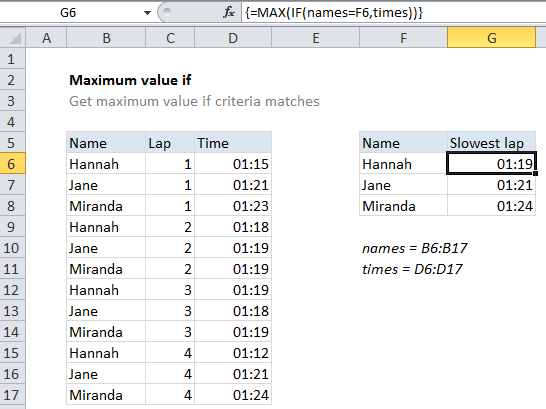## Excel Office

Excel How Tos, Tutorials, Tips & Tricks, Shortcuts

# Maximum value if in Excel

This tutorial shows how to calculate Maximum value if in Excel using the example below;

## Formula

`{=MAX(IF(range=criteria,values))}`## Explanation

To get a maximum value based on criteria, you can use the MAX function together with the IF function in an array formula. In the example shown, the formula in cell G6 is:

`{=MAX(IF(names=F6,times))}`

Where names is the named range B6:B17, and times is the named range D6:D17.

Worked Example:   Max of every nth column in Excel

Notes: this is an array formula and must be entered with Control + Shift + Enter. Later versions of Excel have a MAXIFS function, see note below.

### How this formula works

The IF function is evaluated first. The logical test names=F6 returns an array of TRUE / FALSE values, where TRUE values correspond to rows where the name matches “Hannah” in F6. The “value if true” for IF is the named range “times”, which returns the full set of times. No “value if false” is provided, so the result of IF is an array that contains a time (where the logical test returned TRUE) and FALSE where it didn’t.

Worked Example:   Calculate shipping cost with VLOOKUP in Excel

The MAX function then returns the maximum value in this array. FALSE values are automatically ignored.

Worked Example:   Split numbers from units of measure in Excel

### With MAXIFS

The MAXIFS function, introduced in Excel 2016 via Office 365 subscription, is designed to calculate maximums based on one or more criteria without the need for an array formula. With MAXIFS, the formula in G6 is:

`=MAXIFS(times,names,F6)`